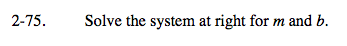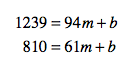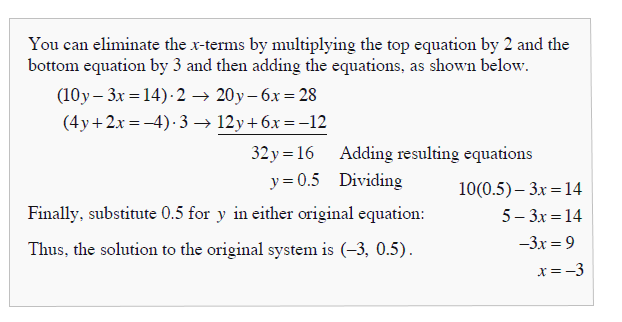Home > A2C > Chapter 2 > Lesson 2.1.5 > Problem2-75

2-75.
1. Solve the system below for m and b. Homework Help ✎

1239 = 94m + b
810 = 61m + bRefer to the Math Notes box from Lesson 2.1.3 (Shown below).

810 = 61(13) + b

810 = 793 + b13 = m

b = 17